《面向对象程序设计基础》课程笔记的主要部分。内含：

• 创建与销毁
• 引用与复制
• 组合与继承
• 虚函数
• 多态与模板
• STL 初步

## Week 05 创建和销毁

### 5.0 Overview

• 5.1 友元
• 5.2 静态成员与常量成员
• 5.3 常量/静态/参数对象的构造与析构时机
• 5.4 对象的new和delete

### 5.1 友元

• 友元

• 被声明为友元的函数或类，具有对出现友元声明的类的private及protected成员的访问权限，即可以访问该类的一切成员。
• 友元的声明只能在类内进行。
• 可以声明别的类的成员函数，包括构造和析构函数，为当前类的友元。

• 友元的声明与当前所在域是否为private或public无关。

### 5.2 静态成员与常量成员

#### 5.2.1 static

1. 静态变量与静态函数
• 静态变量：使用static修饰的变量
• 初始化：初次定义时需要初始化，且只能初始化一次。
• 静态局部变量存储在静态存储区，生命周期将持续到整个程序结束
• 静态全局变量是内部可链接的，作用域仅限其声明的文件，不能被其他文件所用，可以避免和其他文件中的同名变量冲突
• 静态函数：使用static修饰的函数
• 静态函数是内部可链接的，作用域仅限其声明的文件，不能被其他文件所用，可以避免和其他文件中的同名函数冲突
1. 静态数据成员与静态成员函数
• 静态数据成员：使用static修饰的数据成员，是隶属于类的，称为类的静态数据成员，也称“类变量”

• 静态数据成员被该类的所有对象共享（即所有对象中的这个数据域处在同一内存位置）
• 类的静态成员（数据、函数）既可以通过对象来访问，也可以通过类名来访问，如ClassName::static_var或者a.static_var（a为ClassName类的对象）
• 类的静态数据成员要在实现文件中赋初值，格式为：Type ClassName::static_var = Value;
• 和全局变量一样，类的静态数据成员在程序开始前初始化
• 静态成员函数：在返回值前面添加static修饰的成员函数，称为类的静态成员函数

• 和静态数据成员类似，类的静态成员函数既可以通过对象来访问，也可以通过类名来访问，如ClassName::static_function或者a.static_function(a为ClassName类的对象）
• 静态成员函数不能访问非静态成员。（原因：分配时序）

#### 5.2.2 const

1. 常量
• 修饰变量时（如const int n = 1;），必须就地初始化，该变量的值在其生命周期内都不会发生变化
• 修饰引用/指针时（如int a=1; const int& b=a;），不能通过该引用/指针修改相应变量的值，常用于函数参数以保证函数体中无法修改参数的值
• 修饰函数返回值时（如const int* func() {…}），函数返回值的内容（或其指向的内容）不能被修改
1. 常量数据成员和常量成员函数
• 常量数据成员：使用const修饰的数据成员，称为类的常量数据成员，在对象的整个生命周期里不可更改

• 常量数据成员可以在
• 构造函数的初始化列表中被初始化
• 就地初始化
• 不允许在构造函数的函数体中通过赋值来设置
• 常量成员函数

• 成员函数也能用const来修饰，称为常量成员函数。
• 常量成员函数的访问权限：实现语句不能修改类的数据成员，即不能改变对象状态（内容）
ReturnType Func(…) const {…}
• 注意区别：const ReturnType Func(…) {…}
• 若对象被定义为常量(const ClassName a;)，则它只能调用以const修饰的成员函数
• 常量对象：对象中的“数据”不能变
1. 常量静态变量
• 当然，我们可以定义既是常量也是静态的变量
• 作为类的常量变量
• 常量静态变量需要在类外进行定义，但有两个例外：int和enum类型可以就地初始化
• 常量静态变量和静态变量一样，满足访问权限的任意函数均可访问，但都不能修改
class foo {
static const char* cs; // 不可就地初始化
static const int i = 3; // 可以就地初始化
static const int j; // 也可以在类外定义
};

const char* foo::cs = "foo C string";
const int foo::j = 4;

### 5.3 常量/静态/参数对象的构造与析构时机

#### 5.3.1 常量对象的构造与析构

• 常量全局/局部对象的构造与析构时机和普通全局/局部对象相同
• 常量全局对象：在main()函数调用之前进行初始化，在main()函数执行完return，程序结束时，对象被析构
常量局部对象：在程序执行到该局部对象的代码时被初始化。在局部对象生命周期结束、即所在作用域结束后被析构

#### 5.3.2 静态对象的构造与析构

1. 静态全局对象
• 静态全局对象的构造与析构时机和普通全局对象相同
1. 函数中静态对象
• 函数内部定义的静态局部对象

• 在程序执行到该静态局部对象的代码时被初始化，离开作用域不析构。

• 第二次执行到该对象代码时，不再初始化，直接使用上一次的对象。
• 在main()函数结束后被析构。
1. 类静态对象
• 类A的对象a作为类B的静态变量
• a的构造与析构表现和全局对象类似，即在main()函数调用之前进行初始化，在main()函数执行完return，程序结束时，对象被析构
• 和B是否实例化无关

## Week 06 引用与复制

• 6.1 常量引用
• 6.2 拷贝构造函数
• 6.3 右值引用
• 6.4 移动构造函数
• 6.5 赋值运算符
• 6.6 类型转换

### 6.1 常量引用

• 最小特权原则：给函数足够的权限去完成相应的任务，但不要给予他多余的权限。
• 例如函数void add(int& a, int& b)，如果将参数类型定义为int&，则给予该函数在函数体内修改a和b的值的权限
• 如果我们不想给予函数修改权限，则可以在参数中使用常量/常量引用
• void add(const int& a, const int& b)
• 此时函数中仅能读取a和b的值，无法对a, b进行任何修改操作。

### 6.2 拷贝构造函数

• 拷贝构造函数是一种特殊的构造函数，它的参数是语言规定的，是同类对象的常量引用
• MyClass(const MyClass&) {}
• 拷贝构造函数被调用的三种常见情况
• 用一个类对象定义另一个新的类对象
• 函数调用时以类的对象为形参
• 函数返回类对象
// Example 6.2.1

// a
Test a; // NO
Test b(a); //YES
Test c = a; //YES

// b
Func(Test a)

// c
return a;
• 如果调用拷贝构造函数且当前没有给类显式定义拷贝构造函数，编译器将自动合成“隐式定义的拷贝构造函数”，其功能是调用所有数据成员的拷贝构造函数或拷贝赋值运算符
• 隐式定义拷贝构造函数在遇到指针类型成员时可能会出错,导致多个指针类型的变量指向同一个地址。
• 拷贝构造函数的频繁调用会降低程序运行的效率，解决方法：
• 使用引用/常量引用来传参或返回对象
• 将拷贝构造函数声明为 private ，或使用 delete 取消拷贝构造函数的隐式合成

### 6.3 右值引用

• 左值和右值
• 左值：可以取地址、有名字的值。
• 右值：不能取地址、没有名字的值; 常见于常值、函数返回值、表达式
// Example 6.3.1
int a = 1;
int b = func();
int c = a + b;
// 其中a、b、c为左值，1、func函数返回值、a+b的结果为右值。
• 右值引用
• 虽然右值无法取地址，但可以被&&引用(右值引用)
• int &&e = a+b;
• 右值引用无法绑定左值
• int &&e = a; //Compile Error
• 例外：常量左值引用能也绑定右值
• const int &e = 3;

### 6.4 移动构造函数

• 移动构造函数
• 右值引用可以延续即将销毁变量的生命周期，用于构造函数可以提升处理效率，在此过程中尽可能少地进行拷贝。
• 使用右值引用作为参数的构造函数叫做移动构造函数。
// Example 6.4.1

// 拷贝构造函数
ClassName(const ClassName& VariableName);
// 移动构造函数
ClassName(ClassName&& VariableName);
• 移动构造函数与拷贝构造函数最主要的差别就是类中堆内存是重新开辟并拷贝，还是直接将指针指向那块地址。
• 对于一些即将析构的临时类，移动构造函数直接利用了原来临时对象中的堆内存，新的对象无需开辟内存，临时对象无需释放内存，从而大大提高计算效率。
// Example 6.4.2

class Test {
public:
int * buf; //// only for demo.
Test() {
buf = new int; //申请一块内存
cout << "Test(): this->buf @ " << hex << buf << endl;
}
~Test() {
cout << "~Test(): this->buf @ " << hex << buf << endl;
if (buf) delete[] buf;
}
Test(const Test& t) : buf(new int) {
for(int i=0; i<10; i++)
buf[i] = t.buf[i]; //拷贝数据
cout << "Test(const Test&) called. this->buf @ "
<< hex << buf << endl;
}
Test(Test&& t) : buf(t.buf) { //直接复制地址，避免拷贝
cout << "Test(Test&&) called. this->buf @ "
<< hex << buf << endl;
t.buf = nullptr; //将t.buf改为nullptr，使其不再指向原来内存区域
}
};

Test GetTemp() {
Test tmp;
cout << "GetTemp(): tmp.buf @ "
<< hex << tmp.buf << endl;
return tmp;
}

void fun(Test t) {
cout << "fun(Test t): t.buf @ "
<< hex << t.buf << endl;
}

int main() {
Test a = GetTemp();
cout << "main() : a.buf @ " << hex << a.buf << endl;
fun(a);
return 0;
}

// g++ test.cpp --std=c++11 -fno-elide-constructors -o test
• 移动语义
• std::move 函数
• 输入：左值（包括变量等，该左值一般不再使用）
• 返回值：该左值对应的右值

### 6.5 赋值运算符

• 拷贝复制运算符
// Example 6.5.1

// 区分
ClassName a;
ClassName b;
a = b;

ClassName a = b;

// 前者调用
ClassName& operator= (const ClassName& right) {
if (this != &right) {// 避免自己赋值给自己
// 将right对象中的内容拷贝到当前对象中...
}
return *this;
}
• 移动赋值运算符
// Example 6.5.2
Test& operator= (Test&& right) {
if (this == &right)  cout << "same obj!\n";
else {
this->buf = right.buf;  //直接赋值地址
right.buf = nullptr;
cout << "operator=(Test&&) called.\n";
}
return *this;
}

### 6.6 类型转换

1. 在源类中定义目标类型转换运算符
// Example 6.6.1
#include <iostream>
using namespace std;

class Dst { //目标类Destination
public:
Dst() { cout << "Dst::Dst()" << endl; }
};

class Src { //源类Source
public:
Src() { cout << "Src::Src()" << endl; }
operator Dst() const {
cout << "Src::operator Dst() called" << endl;
return Dst();
}
};
1. 在目标类中定义“源类对象作参数的构造函数”
// Example 6.6.2
#include <iostream>
using namespace std;

class Src;	// 前置类型声明，因为在Dst中要用到Src类
class Dst {
public:
Dst() { cout << "Dst::Dst()" << endl; }
Dst(const Src& s) {
cout << "Dst::Dst(const Src&)" << endl;
}
};

class Src {
public:
Src() { cout << "Src::Src()" << endl; }
};
• 注意：两种自动类型转换的方法不能同时使用，使用时请任选其中一种。

• 禁止自动类型转换

• 如果用 explicit 修饰类型转换运算符或类型转换构造函数，则相应的类型转换必须显式地进行

## Week 08 组合与继承

• 组合
• 继承
• 成员访问权限
• 重写隐藏与重载
• 多重继承

### 8.1 组合

• 对象组合的两种实现方法：
• 已有类的对象作为新类的公有数据成员，这样通过允许直接访问子对象而“提供”旧类接口
• 已有类的对象作为新类的私有数据成员。新类可以调整旧类的对外接口，可以不使用旧类原有的接口（相当于对接口作了转换）
• 对象拷贝与赋值运算
• 如果调用拷贝构造函数且没有给类显式定义拷贝构造函数，编译器将自动合成：
• 对有显式定义拷贝构造函数的子对象调用该拷贝构造函数
• 对无显式定义拷贝构造函数的子对象采用位拷贝
• 赋值的默认操作类似

### 8.2 继承

• 基本概念

• 被继承的已有类，被称为基类 base class，也称“父类”。
• 通过继承得到的新类，被为派生类 derived class，也称“子类”、“扩展类”。
• 继承方式

• 常见的继承方式：public, private
• class Derived : [private] Base { .. }; 缺省继承方式
• class Derived : public Base { ... };
• protected 继承很少被使用
• class Derived : protected Base { ... };
• 什么不能被继承？

• 构造函数：创建派生类对象时，必须调用派生类的构造函数，派生类构造函数调用基类的构造函数，以创建派生对象的基类部分。C++11新增了继承构造函数的机制（使用using），但默认不继承
• 析构函数：释放对象时，先调用派生类析构函数，再调用基类析构函数
• 赋值运算符：因为赋值运算符包含一个类型为其所属类的形参
• 友元函数：不是类成员
• 派生类对象的构造与析构过程
• 调用基类构造函数

• 若没有显式调用，则编译器会自动生成一个对基类的默认构造函数的调用。
• 若想要显式调用，则只能在派生类构造函数的初始化成员列表中进行。
• 继承基类构造函数

• 在派生类中使用 using Base::Base; 来继承基类构造函数，相当于给派生类“定义”了相应参数的构造函数，如下例 8.2.1.
• 当基类存在多个构造函数时，使用using会给派生类自动构造多个相应的构造函数，如下例 8.2.2.
• 如果基类的某个构造函数被声明为私有成员函数，则不能在派生类中声明继承该构造函数。
• 如果派生类使用了继承构造函数，编译器就不会再为派生类生成默认构造函数。
// Example 8.2.1
class Base
{
int data;
public:
Base(int i) : data(i) { cout << "Base::Base(" << i << ")\n"; }
};

class Derive : public Base {
public:
using Base::Base; 		///相当于 Derive(int i):Base(i){};
};

int main() {
Derive obj(356);

return 0;
} 
// Example 8.2.2
class Base
{
int data;
public:
Base(int i) : data(i) { cout << "Base::Base(" << i << ")\n"; }
Base(int i, int j)
{ cout << "Base::Base(" << i << “," << j << ")\n";}
};

class Derive : public Base {
public:
using Base::Base; 		///相当于 Derive(int i):Base(i){};
///加上 Derive(int i, int j):Base(i，j){};
};

int main() {
Derive obj(356);
Derive obj(356, 789);
return 0;
}
• 继承方式
• public 继承：基类中公有成员仍能在派生类中保持公有。（图 8.2.3）
• private 继承：用基类接口实现派生类功能。（图 8.2.4）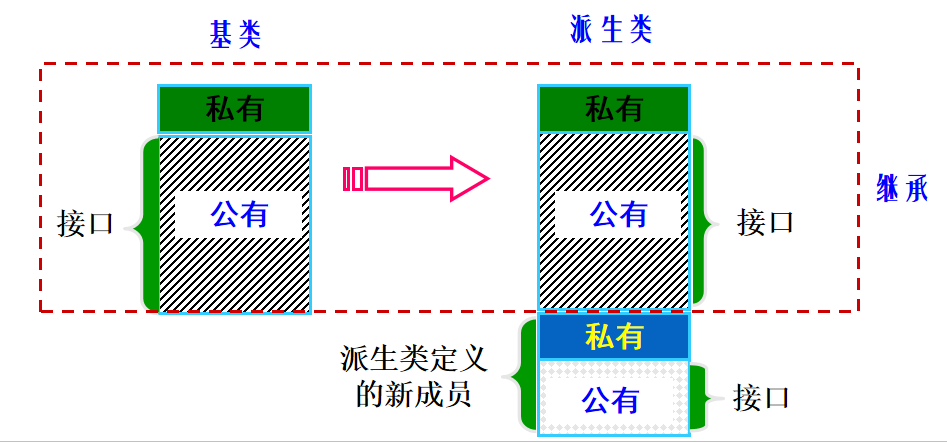（图 8.2.3）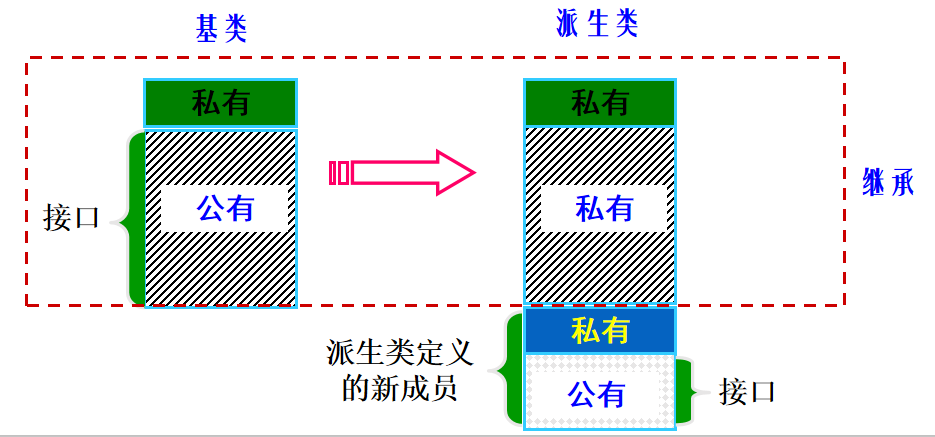（图 8.2.4）

### 8.3 成员访问权限

• 基类中的私有成员
• 不允许在派生类成员函数中访问
• 不允许派生类的对象访问它们
• 基类中的公有成员
• 允许在派生类成员函数中被访问
• 若是使用public继承方式，则成为派生类公有成员，可以被派生类的对象访问
• 若是使用 private/protected 继承方式，则成为派生类私有/保护成员，不能被派生类的对象访问
• 若想让某成员能被派生类的对象访问，可在派生类 public 部分用关键字 using 声明它的名字（例 8.3.1）
• 基类中的保护成员
• 与基类中的私有成员的不同在于：保护成员允许在派生类成员函数中被访问。
// Example 8.3.1
#include <iostream>
using namespace std;
class Base {
public:
void baseFunc() { cout << "in Base::baseFunc()..." << endl; }
};

class Derive3: private Base {// B的私有继承
public:
/// 私有继承时，在派生类public部分声明基类成员名字
using Base::baseFunc;
};

int main() {
Derive3 obj3;
cout << "calling obj3.baseFunc()..." << endl;
obj3.baseFunc(); //基类接口在派生类public部分声明，则派生类对象可调用

return 0;
}
• 基类成员的访问权限
• public 继承：基类的公有成员，保护成员，私有成员作为派生类的成员时，都保持原有的状态。
• private 继承：基类的公有成员，保护成员，私有成员作为派生类的成员时，都作为私有成员。
• protected 继承：基类的公有成员，保护成员作为派生类的成员时，都成为保护成员，基类的私有成员仍然是私有的。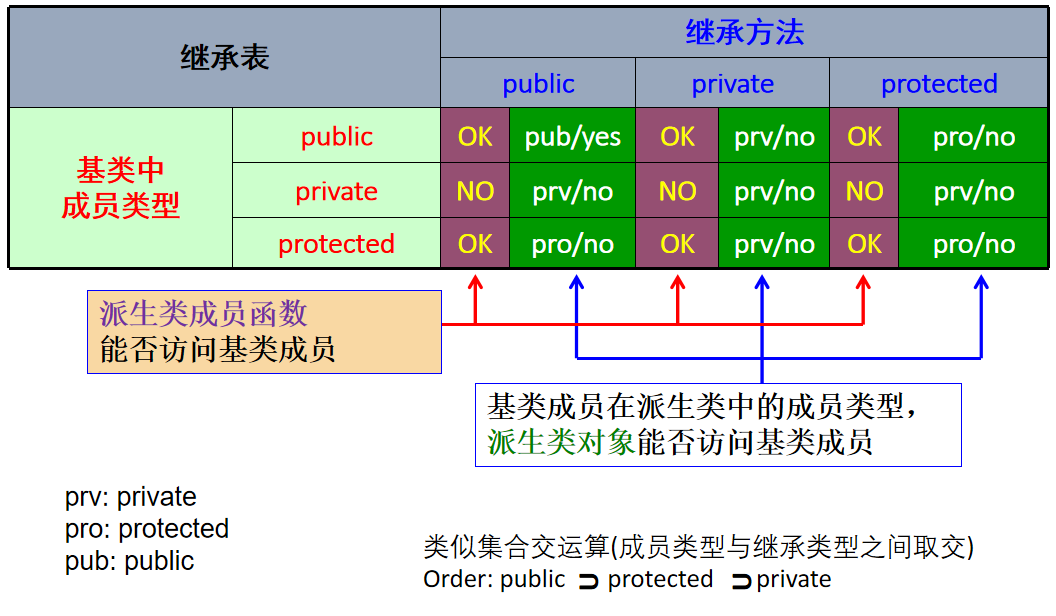（表 8.3.2）

### 8.4 重写隐藏与重载

• 目的：提供同名函数的不同实现，属于静态多态。
• 函数名必须相同，函数参数必须不同，作用域相同（如位于同一个类中；或同名全局函数）。
• 重写隐藏(redefining)：
• 目的：在派生类中重新定义基类函数，实现派生类的特殊功能。
• 屏蔽了基类的所有其它同名函数。（例 8.4.1）
• 函数名必须相同，函数参数可以不同
• 可以在派生类中通过 using 类名::成员函数名; 在派生类中“恢复”指定的基类成员函数（即去掉屏蔽），使之重新可用（例 8.4.2）

// Example 8.4.1
#include <iostream>
using namespace std;

class T {};

class Base {
public:
void f() { cout << "B::f()\n"; }
void f(int i) { cout << "Base::f(" << i << ")\n"; } /// 重载
void f(double d) { cout << "Base::f(" << d << ")\n"; } ///重载
void f(T) { cout << "Base::f(T)\n"; } ///重载
};

class Derive : public Base {
public:
void f(int i) { cout << "Derive::f(" << i << ")\n"; } ///重写隐藏
};

int main() {
Derive d;
d.f(10);
d.f(4.9);		/// 编译警告。执行自动类型转换。
//  d.f();		/// 被屏蔽，编译错误
//  d.f(T());	/// 被屏蔽，编译错误
return 0;
}
// Example 8.4.2
#include <iostream>
using namespace std;

class T {};

class Base {
public:
void f() { cout << "Base::f()\n"; }
void f(int i) { cout << "Base::f(" << i << ")\n"; }
void f(double d) { cout << "Base::f(" << d << ")\n"; }
void f(T) { cout << "Base::f(T)\n"; }
};

class Derive : public Base {
public:
using Base::f;
void f(int i) { cout << "Derive::f(" << i << ")\n"; }
};

int main() {
Derive d;
d.f(10);
d.f(4.9);
d.f();
d.f(T());
return 0;
}
• using 关键字
• 继承基类构造函数
• 恢复被屏蔽的基类成员函数
• 还可用于：
• 指示命名空间，using namespace std;
• 将另一个命名空间的成员引入当前命名空间using std::cout; cout << endl;
• 定义类型别名，using a = int;

### 8.5 多重继承

• 派生类同时继承多个基类
class File{};
class InputFile: public File{};
class OutputFile: public File{};
class IOFile: public InputFile, public OutputFile{};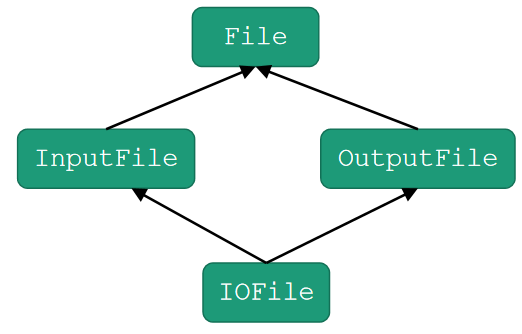（图 8.5.1）

• 数据存储
• 如果派生类D继承的两个基类A,B，是同一基类Base的不同继承，则A,B中继承自Base的数据成员会在D有两份独立的副本，可能带来数据冗余。
• 二义性
• 如果派生类D继承的两个基类A,B，有同名成员a，则访问D中a时，编译器无法判断要访问的哪一个基类成员。
// Example 8.5.2
#include <iostream>
using namespace std;

class Base {
public:
int a{0};
};

class MiddleA : public Base {
public:
void addA() { cout << "a=" << ++a << endl; };
void bar() { cout << "A::bar" << endl; };
};

class MiddleB : public Base {
public:
void addB() { cout << "a=" << ++a << endl; };
void bar() { cout << "B::bar" << endl; };
};

class Derive : public MiddleA, public MiddleB{
};

int main() {
Derive d;
cout << d.a; 	/// 编译错误，A和B都有成员a
cout << d.A::a; /// 输出A中的成员a的值
d.bar(); 		/// 编译错误，A和B都有成员函数bar
return 0;
}

## Week 09 虚函数

### 9.0 Overview

• 向上类型转换
• 对象切片
• 函数调用捆绑
• 虚函数和虚函数表
• 虚函数和构造函数、析构函数
• 重写覆盖，override和final

### 9.1 向上类型转换

• 派生类对象/引用/指针转换成基类对象/引用/指针，称为向上类型转换。只对public继承有效，在继承图上是上升的；对privateprotected继承无效。
• 向上类型转换（派生类到基类）可以由编译器自动完成，是一种隐式类型转换。
• 凡是接受基类对象/引用/指针的地方（如函数参数），都可以使用派生类对象/引用/指针，编译器会自动将派生类对象转换为基类对象以便使用。

### 9.2 对象切片

• 派生类的对象(不是指针或引用)被通过传参或赋值的方式转换为基类的对象时，派生类的对象被切片为对应基类的子对象。

•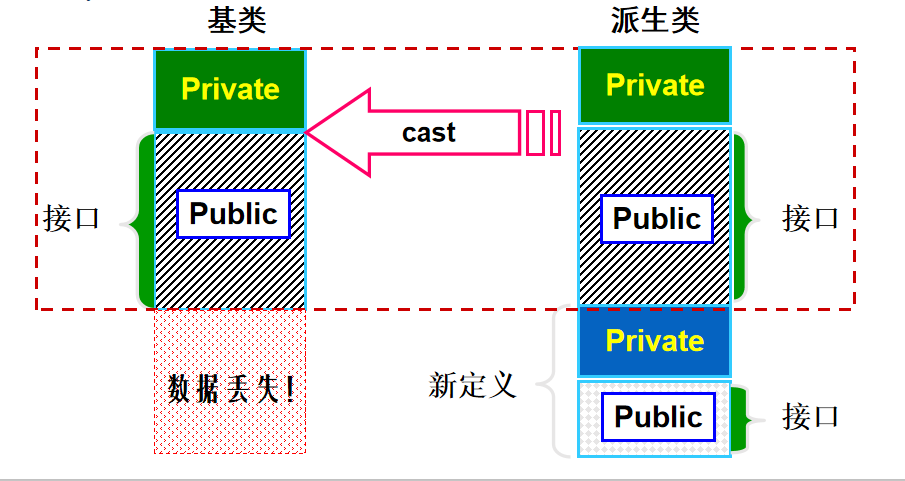• 派生类的新数据和新方法丢失（图 9.2.1）
• 当派生类的指针（引用）被通过传参或赋值的方式转换为基类指针（引用）时，不会创建新的对象，但只保留基类的接口。

// Example 9.2.2 私有继承“照此实现”
#include <iostream>
using namespace std;
class B {
private:
int data{0};
public:
int getData() { return data; }
void setData(int i) { data = i; }
};
class D1 : private B {
public:
using B::getData;
};
int main() {
D1 d1;
cout << d1.getData();
// d1.setData(10) //隐藏了基类的setData函数，不可访问
// B& b = d1;     //不允许私有继承的向上转换
// b.setData(10); //否则可以绕过D1，调用基类的setData函数
}

### 9.3 函数调用捆绑

// Example 9.3.1
#include <iostream>
using namespace std;

class Instrument {
public:
void play() { cout << "Instrument::play" << endl; }
};

class Wind : public Instrument {
public:
// Redefine interface function:
void play() { cout << "Wind::play" << endl; }
};

void tune(Instrument& i) {
i.play();
}

int main() {
Wind flute;
tune(flute); //引用的向上类型转换(传参)，编译器早绑定，无对象切片产生
Instrument &inst = flute;  // 引用的向上类型转换(赋值)
inst.play();
}
• 把函数体与函数调用相联系称为捆绑(binding)。
• 即将函数体实现代码的入口地址，与调用的函数名绑定。执行到调用代码时进入函数体内部。
• 当捆绑在程序运行之前（由编译器和连接器）完成时，称为早捆绑(early binding)。
• 运行之前已经决定了函数调用代码到底进入哪个函数。
• 上面程序中的问题是早捆绑引起的，编译器将tune中的函数调用i.play()与Instrument::play()绑定。
• 当捆绑根据对象的实际类型(上例中即子类Wind而非Instrument)，发生在程序运行时，称为晚捆绑(late binding)，又称动态捆绑或运行时捆绑。
• 要求在运行时能确定对象的实际类型，并绑定正确的函数。
• 晚捆绑只对类中的虚函数起作用，使用 virtual 关键字声明虚函数。

### 9.4 虚函数与虚函数表

• 对于被派生类重新定义的成员函数，若它在基类中被声明为虚函数，则通过基类指针或引用调用该成员函数时，编译器将根据所指（或引用）对象的实际类型决定是调用基类中的函数，还是调用派生类重写的函数。
• 若某成员函数在基类中声明为虚函数，当派生类重写覆盖(同名，同参数函数)它时，无论是否声明为虚函数，该成员函数都仍然是虚函数。
// Example 9.4.1
#include <iostream>
using namespace std;

class Instrument {
public:
virtual void play() { cout << "Instrument::play" << endl; }
};

class Wind : public Instrument {
public:
void play() { cout << "Wind::play" << endl; }
/// 重写覆盖(稍后：重写隐藏和重写覆盖的区别）
};

void tune(Instrument& ins) {
ins.play(); /// 由于 Instrument::play 是虚函数，编译时不再直接绑定，运行时根据 ins 的实际类型调用。
}

int main() {
Wind flute;
tune(flute); /// 向上类型转换
}

• 一般来说，派生类虚函数的返回类型应该和基类相同。

• 或者，是协变(Covariant)的，例如

• 基类和派生类的指针是协变的
• 基类和派生类的引用是协变的
• cpp
// Example 9.4.2

# include

using namespace std;

class Instrument {
public:
virtual Instrument& getObj() { return *this; }
};

class Wind : public Instrument {
public:
virtual Wind& getObj() { return *this;}
//Wind&和Instrument&协变
};


+ 虚函数表
+ 对象自身要包含自己实际类型的信息：用虚函数表表示。运行时通过虚函数表确定对象的实际类型。
+ **虚函数表**(VTABLE)：每个包含虚函数的类用于存储虚函数地址的表(虚函数表有唯一性，即使没有重写虚函数)。
+ 每个**包含虚函数的类对象**中，编译器秘密地放一个**指针**，称为**虚函数指针**(vpointer/VPTR)，指向这个类的VTABLE。
+ 当通过基类指针做虚函数调用时，编译器静态地插入能取得这个VPTR并在VTABLE表中查找函数地址的代码，这样就能调用正确的函数并引起晚捆绑的发生。
+ **编译**期间：**建立虚函数表VTABLE**，记录每个类或该类的基类中所有已声明的虚函数入口地址。
+ **运行**期间：**建立虚函数指针VPTR**，在构造函数中发生，指向相应的VTABLE。

cpp
// Example 9.4.3
#include <iostream>
using namespace std;
class B {
public:
virtual void fun1() { cout << "B::fun1()" << endl; }
virtual void fun2() { cout << "B::fun2()" << endl; }

private:
int i;
float j;
};
class D : public B {
public:
virtual void fun1() {
cout << "D::fun1()" << endl;
}  ///对fun1重写覆盖，对fun2没有，则fun2使用基类的虚函数地址
double k;
};
int main() {
B b;
D d;
B* pB = &d;
pB->fun1();
}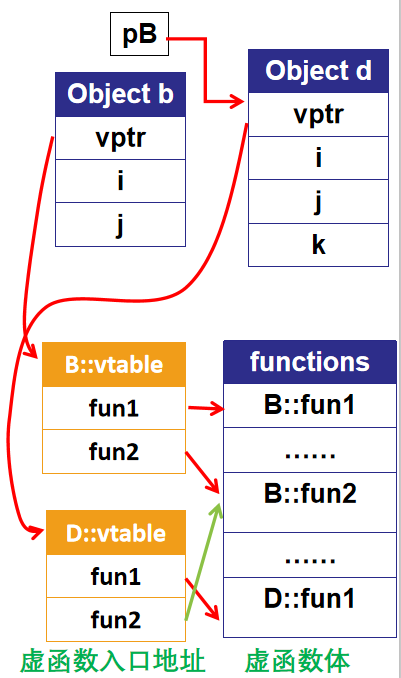（图 9.4.4）

### 9.5 虚函数与构造函数、析构函数

• 虚函数与构造函数

• 当创建一个包含有虚函数的对象时，必须初始化它的VPTR以指向相应的VTABLE。设置VPTR的工作由构造函数完成。编译器在构造函数的开头秘密的插入能初始化VPTR的代码。
• 构造函数不能也不必是虚函数。
• 不能：如果构造函数是虚函数，则创建对象时需要先知道VPTR，而在构造函数调用前，VPTR未初始化。
• 不必：构造函数的作用是提供类中成员初始化，调用时明确指定要创建对象的类型，没有必要是虚函数。
• 在构造函数中调用一个虚函数，被调用的只是这个函数的本地版本(即当前类的版本)，即虚机制在构造函数中不工作。
• 初始化顺序：(与构造函数初始化列表顺序无关)
• 基类初始化
• 对象成员初始化
• 构造函数体
• 原因：基类的构造函数比派生类先执行，调用基类构造函数时派生类中的数据成员还没有初始化。如果允许调用实际对象的虚函数，则可能会用到未初始化的派生类成员。
• 虚函数与析构函数

• 析构函数能是虚的，且常常是虚的。虚析构函数仍需定义函数体。
• 虚析构函数的用途：当删除基类对象指针时，编译器将根据指针所指对象的实际类型，调用相应的析构函数。
• 若基类析构不是虚函数，则删除基类指针所指派生类对象时，编译器仅自动调用基类的析构函数，而不会考虑实际对象是不是基类的对象。这可能会导致内存泄漏。
• 在析构函数中调用一个虚函数，被调用的只是这个函数的本地版本，即虚机制在析构函数中不工作。
// Example 9.5.1
#include <iostream>
using namespace std;

class Base1 {
public:
~Base1() { cout << "~Base1()\n"; }
};

class Derived1 : public Base1 {
public:
~Derived1() { cout << "~Derived1()\n"; }
};

class Base2 {
public:
virtual ~Base2() { cout << "~Base2()\n"; }
};

class Derived2 : public Base2 {
public:
~Derived2() { cout << "~Derived2()\n"; }
};

int main() {
Base1* bp = new Derived1;
delete bp; /// 只调用了基类的虚析构函数
Base2* b2p = new Derived2;
delete b2p; /// 派生类虚析构函数调用完后调用基类的虚析构函数
}

// Output
~Base1()
~Derived2()
~Base2()
• 重要原则：总是将基类的析构函数设置为虚析构函数

### 9.6 重载、重写覆盖与重写隐藏

• 函数名必须相同，函数参数必须不同，作用域相同(同一个类)，返回值可以相同或不同。
• 重写覆盖(override)：
• 派生类重新定义基类中的虚函数函数名必须相同，函数参数必须相同返回值一般情况应相同
• 派生类的虚函数表中原基类的虚函数指针会被派生类中重新定义的虚函数指针覆盖掉。
• 重写隐藏(redefining)：
• 派生类重新定义基类中的函数，函数名相同，但是参数不同或者基类的函数不是虚函数
• 虚函数表不会发生覆盖。
• 重写覆盖和重写隐藏：

• 相同点：
• 都要求派生类定义的函数与基类同名。
• 都会屏蔽基类中的同名函数，即派生类的实例无法调用基类的同名函数。
• 不同点：
• 重写覆盖要求基类的函数是虚函数，且函数参数相同，返回值一般情况应相同；重写隐藏要求基类的函数不是虚函数或者函数参数不同。
• 重写覆盖会使派生类虚函数表中基类的虚函数的指针被派生类的虚函数指针覆盖。重写隐藏不会。
• override与final关键字

• 重写覆盖要满足的条件很多，很容易写错，可以使用override关键字辅助检查。
• override关键字明确地告诉编译器一个函数是对基类中一个虚函数的重写覆盖，编译器将对重写覆盖要满足的条件进行检查，正确的重写覆盖才能通过编译。
• 如果没有override关键字，但是满足了重写覆盖的各项条件，也能实现重写覆盖。它只是编译器的一个检查，正确实现override时，对编译结果没有影响。
• 不想让使用者继承？-> final关键字!

• 在虚函数声明或定义中使用时，final确保函数为虚且不可被派生类重写。可在继承关系链的“中途”进行设定，禁止后续派生类对指定虚函数重写。
• 在类定义中使用时，final指定此类不可被继承。

## Week 10 多态与模板

### 10.0 Overview

• 纯虚函数与抽象类
• 向下类型转换
• 多重继承的虚函数表，多重继承的利弊
• 多态
• 函数模板与类模板

### 10.1 纯虚函数与抽象类

• 虚函数还可以进一步声明为纯虚函数，包含纯虚函数的类，通常被称为“抽象类”。

• virtual 返回类型 函数名(形式参数) = 0;
• 抽象类不允许定义对象，定义基类为抽象类的主要用途是为派生类规定共性“接口”

// Example 10.1.1
class A {
public:
virtual void f() = 0; /// 可在类外定义函数体提供默认实现。派生类通过 A::f() 调用
};
A obj; /// 不准抽象类定义对象！编译不通过！
• 抽象类

• 定义：含有至少一个纯虚函数。
• 特点：
• 不允许定义对象。
• 只能为派生类提供接口。
• 能避免对象切片：保证只有指针和引用能被向上类型转换。
• 基类纯虚函数被派生类重写覆盖之前仍是纯虚函数。因此当继承一个抽象类时，必须实现所有纯虚函数，否则继承出的类也是抽象类。

• 纯虚析构函数除外
• 对于纯虚析构函数而言，即便派生类中不显式实现，编译器也会自动合成默认析构函数。因此，即使派生类不覆盖纯虚析构函数，派生类可以不是抽象类，可以定义派生类对象。
• 回顾：虚函数与析构函数
• 析构函数能是虚的，且常常是虚的。虚析构函数仍需定义函数体
• 虚析构函数的用途：当删除基类对象指针时，编译器将根据指针所指对象的实际类型，调用相应的析构函数。
• 析构函数也可以是纯虚函数
• 纯虚析构函数仍然需要函数体
• 目的：使基类成为抽象类，不能创建基类的对象。如果有其他函数是纯虚函数，则析构函数不必是纯虚的。
// Example 10.1.2
class Base {
public:
virtual ~Base()=0;
};
Base::~Base() {} /// 必须有函数体
class Derive : public Base {};
int main() {
Base b; /// 编译错误，基类是抽象类
Derive d1;
return 0;
}

### 10.2 向下类型转换

• 基类指针/引用转换成派生类指针/引用，则称为向下类型转换。（类层次中向下移动）
• 如何确保转换的正确性？
• 如何保证基类指针指向的对象也可以被要转换的派生类的指针指向？—— 借助虚函数表进行动态类型检查！
• C++提供了一个特殊的显式类型转换，称为dynamic_cast，是一种安全类型向下类型转换。
• 使用dynamic_cast的对象必须有虚函数，因为它使用了存储在虚函数表中的信息判断实际的类型。使用方法：
• obj_p，obj_r分别是T1类型的指针和引用
• T2* pObj = dynamic_cast<T2*>(obj_p);//转换为T2指针，运行时失败返回 nullptr
• T2& refObj = dynamic_cast<T2&>(obj_r); //转换为T2引用，运行时失败抛出 bad_cast 异常
• T1必须是多态类型（声明或继承了至少一个虚函数的类），否则不过编译；T2不必。T1,T2没有继承关系也能通过编译，只不过运行时会转换失败。
• 如果我们知道正在处理的是哪些类型，可以使用static_cast来避免这种开销。
• static_cast在编译时静态浏览类层次，只检查继承关系。没有继承关系的类之间，必须具有转换途径才能进行转换（要么自定义，要么是语言语法支持），否则不过编译。运行时无法确认是否正确转换。
• static_cast使用方法：
• obj_p，obj_r分别是T1类型的指针和引用
• T2* pObj = static_cast<T2*>(obj_p); //转换为T2指针
• T2& refObj = static_cast<T2&>(obj_r); //转换为T2引用
• 不安全：不保证转换后的目标是T2类型的，可能导致非法内存访问。
// Example 10.2.1
#include <iostream>
using namespace std;
class B {
public:
virtual void f(){};
};
class D : public B {
public:
int i{2018};
};
int main() {
D d;
B b;
//    D d1 = static_cast<D>(b); ///未定义类型转换方式
//    D d2 = dynamic_cast<D>(b); ///只允许指针和引用转换

D* pd1 = static_cast<D*>(&b);  /// 有继承关系，允许转换
if (pd1 != nullptr) {
cout << "static_cast, B*(B) --> D*: OK" << endl;
cout << "D::i=" << pd1->i << endl;
}
/// 但是不安全：对D中成员i可能非法访问

D* pd2 = dynamic_cast<D*>(&b);
if (pd2 == nullptr)  /// 不允许不安全的转换
cout << "dynamic_cast, B*(B) --> D*: FAILED" << endl;
}

>>> static_cast, B*(B) --> D*:OK
>>> D::i=124455624
>>> dynamic_cast, B*(B) --> D*: FAILED
// Example 10.2.2
#include <iostream>
using namespace std;
class B {
public:
virtual void f(){};
};
class D : public B {
public:
int i{2018};
};
int main() {
D d;
B b;
//    D d1 = static_cast<D>(b); ///未定义类型转换
//    D d2 = dynamic_cast<D>(b); ///只允许指针和引用转换
B* pb = &d;
D* pd3 = static_cast<D*>(pb);
if (pd3 != nullptr) {
cout << "static_cast, B*(D) --> D*: OK" << endl;
cout << "D::i=" << pd3->i << endl;
}
D* pd4 = dynamic_cast<D*>(pb);
if (pd4 != nullptr) {
cout << "dynamic_cast, B*(D) --> D*: OK" << endl;
cout << "D::i=" << pd4->i << endl;
}
return 0;
}

>>> static_cast, B*(D) --> D*: OK
>>> D::i=2018
>>> dynamic_cast, B*(D) --> D*: OK
>>> D::i=2018
• 重要原则(清楚指针所指向的真正对象)：
1）指针或引用的向上转换总是安全的；
2）向下转换时用dynamic_cast，安全检查；
3）避免对象之间的转换。

• 对于基类中有虚函数的情况：

• 向上类型转换：
• 转换为基类指针或引用，则对应虚函数表仍为派生类的虚函数表（晚绑定）。
• 转换为基类对象，则对应虚函数表是基类的虚函数表（早绑定）。
• 向下类型转换：
dynamic_cast通过虚函数表来判断是否能进行向下类型转换。
// Example 10.2.3
#include <iostream>
using namespace std;

class Pet {
public:
virtual ~Pet() {}
};
class Dog : public Pet {
public:
void run() { cout << "dog run" << endl; }
};
class Bird : public Pet {
public:
void fly() { cout << "bird fly" << endl; }
};

void action(Pet* p) {
auto d = dynamic_cast<Dog*>(p);   /// 向下类型转换
auto b = dynamic_cast<Bird*>(p);  /// 向下类型转换
if (d)  /// 运行时根据实际类型表现特性
d->run();
else if (b)
b->fly();
}

int main() {
Pet* p;
p = new Dog;   /// 向上类型转换
p = new Bird;  /// 向上类型转换
for (int i = 0; i < 2; ++i) {
action(p[i]);
}
return 0;
}

### 10.3 多重继承的虚函数表与利弊

• 多重继承中的虚函数
• 最多继承一个非抽象类 避免 多重继承的二义性
• 可以集成多个抽象类 利用 一个对象可以实现多个接口

### 10.4 多态

• 按照基类的接口定义，调用指针或引用所指对象的接口函数，函数执行过程因对象实际所属派生类的不同而呈现不同的效果（表现），这个现象被称为“多态”。

• 当利用基类指针/引用调用函数时
• 虚函数在运行时确定执行哪个版本，取决于引用或指针对象的真实类型
• 非虚函数在编译时绑定
• 当利用类的对象直接调用函数时
• 无论什么函数，均在编译时绑定
• 产生多态效果的条件：继承 && 虚函数 && (引用 || 指针)
• 应用：TEMPLATE METHOD设计模式

• 在接口的一个方法中定义算法的骨架
• 将一些步骤的实现延迟到子类中
• 使得子类可以在不改变算法结构的情况下，重新定义算法中的某些步骤。
• 模板方法是一种源代码重用的基本技术，在类库的设计实现中应用十分广泛，因为这个设计模式能有效地解决 “类库提供公共行为”与“用户定制特殊细节”之间的折中平衡。
// Example 10.4.1
#include <iostream>
using namespace std;

class Base {
public:
void action() {
step1();
step2();
step3();
}
virtual void step1() { cout << "Base::step1" << endl; }
virtual void step2() { cout << "Base::step2" << endl; }
virtual void step3() { cout << "Base::step3" << endl; }
};

class Derived1 : public Base {
void step1() { cout << "Derived1::step1" << endl; }
};
class Derived2 : public Base {
void step2() { cout << "Derived2::step2" << endl; }
};

int main() {
Base* ba[] = {new Base, new Derived1, new Derived2};
for (int i = 0; i < 3; ++i) {
ba[i]->action();
cout << "===" << endl;
}
return 0;
}

>>>
Base::step1
Base::step2
Base::step3
===
Derived1::step1
Base::step2
Base::step3
===
Base::step1
Derived2::step2
Base::step3
===

### 10.5 函数模板与类模板

1. 函数模板
• 有些算法实现与类型无关，所以可以将函数的参数类型也定义为一种特殊的“参数”，这样就得到了“函数模板”。
• 定义函数模板的方法
• template <typename T> ReturnType Func(Args)；
• 如：任意类型两个变量相加的“函数模板”
• template <typename T> T sum(T a, T b) { return a + b; }
• 注：typename也可换为class
• 函数模板在调用时，编译器能自动推导出实际参数的类型（这个过程叫做实例化）。
• 所以，形式上调用一个函数模板与普通函数没有区别。
• 当多个参数的类型不一致时，无法推导：
• cout << sum(9, 2.1); //编译错误
• 手工指定调用类型：sum<int>(9, 2.1);
1. 类模板
• 在定义类时也可以将一些类型信息抽取出来，用模板参数来替换，从而使类更具通用性。这种类被称为“类模板”。
// Example 10.5.1
#include <iostream>
using namespace std;

template <typename T>
class A {
T data;

public:
void print() { cout << data << endl; }
};
int main() {
A<int> a;
a.print();
}
• 类模板中成员函数的类外定义
// Example 10.5.2
#include <iostream>
using namespace std;

template <typename T>
class A {
T data;

public:
void print();
};

template <typename T>
void A<T>::print() {
cout << data << endl;
}

int main() {
A<int> a;
a.print();
}
• 类模板的“模板参数”
• 类型参数：使用typename或class标记
• 非类型参数：整数，枚举，指针（指向对象或函数），引用（引用对象或引用函数）。整数型比较常用。
// Example 10.5.3
template <typename T, unsigned size>
class array {
T elems[size];
...
};

array<char, 10> array0;
• 模板与多态
• 模板使用泛型标记，使用同一段代码，来关联不同但相似的特定行为，最后可以获得不同的结果。模板也是多态的一种体现。
• 但模板的关联是在编译期处理，称为静多态。
• 往往和函数重载同时使用
• 高效，省去函数调用
• 编译后代码增多
• 基于继承和虚函数的多态在运行期处理，称为动多态
• 运行时，灵活方便
• 侵入式，必须继承
• 存在函数调用

## Week 11 模板与STL初步

### 11.0 Overview

• 类模板与函数模板特化
• 命名空间
• STL初步——容器与迭代器

### 11.1 命名空间

• 为了避免在大规模程序的设计中，以及在程序员使用各种各样的C++库时，标识符的命名发生冲突，标准C++引入了关键字namespace（命名空间），可以更好地控制标识符的作用域。
• 标准C++库（不包括标准C库）中所包含的所有内容（包括常量、变量、结构、类和函数等）都被定义在命名空间std（standard标准）中。
// Example 11.1.1
// 定义命名空间
namespace A {
int x, y;
}
// 使用命名空间
A::x = 3;
A::y = 6;
• 使用using声明简化命名空间使用
• 使用整个命名空间：所有成员都直接可用using namespace A; x = 3; y = 6;
• 使用部分成员：所选成员可直接使用 using A::x; x = 3; A::y = 6;
• 任何情况下，都不应出现命名冲突

### 11.2 STL初步

• 标准模板库（英文：Standard Template Library，缩写：STL），是一个高效的C++软件库，它被容纳于C++ 标准程序库C++ Standard Library中。其中包含4个组件，分别为算法、容器、函数、迭代器。基于模板编写。关键理念：将“在数据上执行的操作”与“要执行操作的数据”分离。

• 简单容器

• 容器是包含、放置数据的工具。通常为数据结构。
• 简单容器（simple container）
• 序列容器（sequence container）
• 关系容器（associative container）
• std::pair
• std::tuple
• std::vector

• 创建：std::vector<int> x;
• 当前数组长度： x.size();
• 清空： x.clear();
• 在末尾添加/删除：（高速）x.push_back(1); x.pop_back();
• 在中间添加/删除：（使用迭代器，低速）x.insert(x.begin()+1, 5); x.erase(x.begin()+1);
• 迭代器

• 一种检查容器内元素并遍历元素的数据类型。
• 提供一种方法顺序访问一个聚合对象中各个元素, 而又不需暴露该对象的内部表示。
• 为遍历不同的聚合结构（需拥有相同的基类）提供一个统一的接口。
• 使用上类似指针。
• 迭代器：失效

• 当迭代器不再指向本应指向的元素时，称此迭代器失效。

• vector中什么情况下会发生迭代器失效？
• 看作纯粹的指针
• 调用insert/erase后，所修改位置之后的所有迭代器失效。（原先的内存空间存储的元素被改变）
• 调用push_back等修改vector大小的方法时，可能会使所有迭代器失效（Push_back到了一定程度之后，可能会造成数组的整体移动，导致所有的内存地址发生改变。）
• std::list

// 插入前端：
l.push_front(1);
// 插入末端：
l.push_back(2);
// 查询：
std::find(l.begin(), l.end(), 2); //返回迭代器
// 插入指定位置：
l.insert(it, 4); //it为迭代器
• std::list

• 不支持下标等随机访问
• 支持高速的在任意位置插入/删除数据
• 其访问主要依赖迭代器
• 操作不会导致迭代器失效（除指向被删除的元素的迭代器外）
• std::set

// 插入：
s.insert(1);
// 查询：
s.find(1);   //返回迭代器
// 删除：
s.erase(s.find(1));   //导致被删除元素的迭代器失效
// 统计：
s.count(1);   //1的个数，总是0或1
• std::map
• 其值类型为pair
• map中的元素key互不相同，需要key存在比较器。
• 可以通过下标访问（即使key不是整数）。下标访问时如果元素不存在，则创建对应元素。